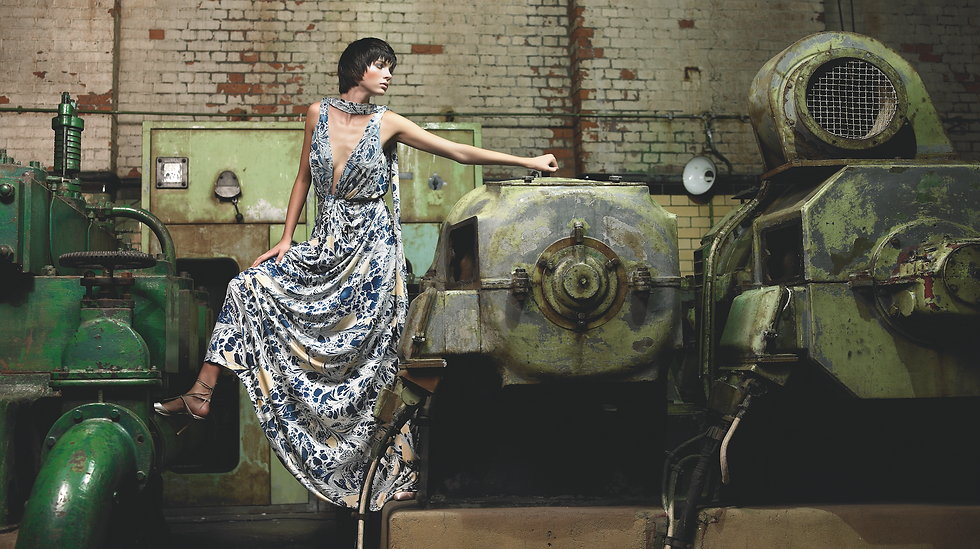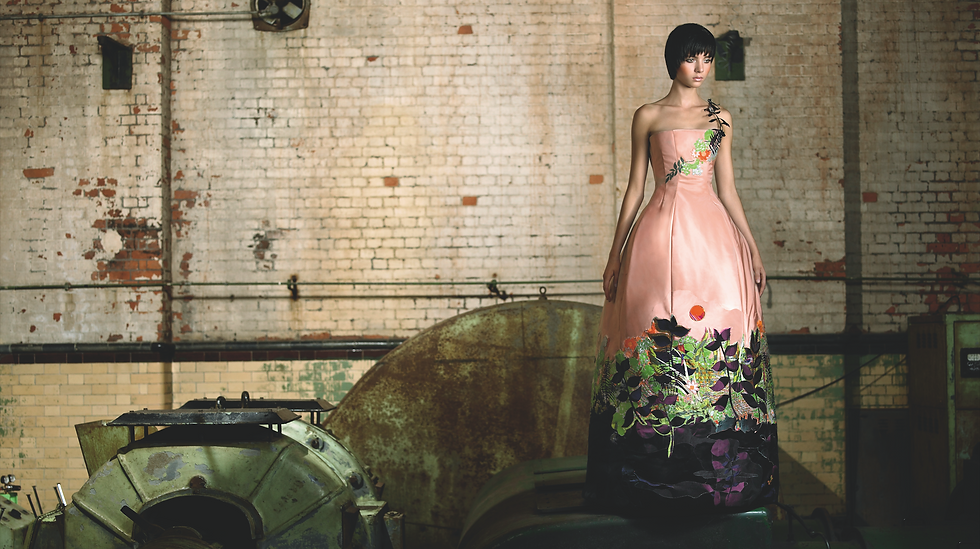top of page

## E C O T U R E ™

Deborah Milner with

A collection of 10 couture dresses which each explore a different aspect of sustainability.Y A W A N A W A   D R E S SB I G   K N O T   D R E S SS M A L L   K N O T   D R E S SS A N D A L W O O D   D R E S SMA R B L E D   D R E S SF I R E W O R K   D R E S SP L A S T I C   L A C E   D R E S SG R E E N   P L E A T   D R E S SA U B E R G I N E   D R E S SR O U S S E A U   D R E S S

bottom of page